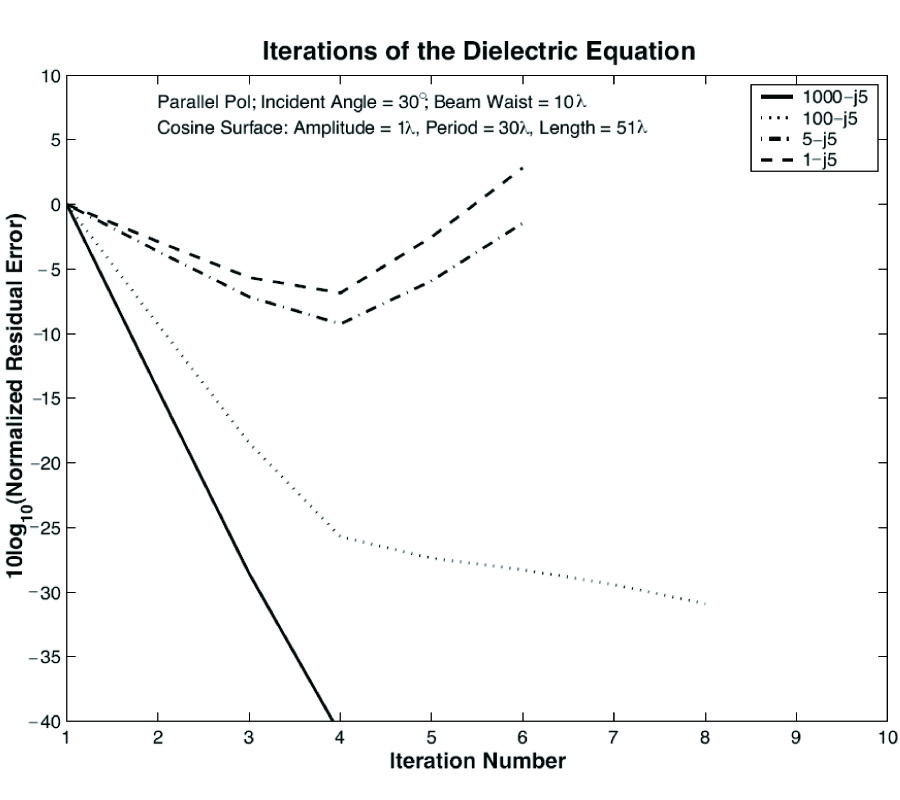# Vol. 42

Front:[PDF file] Back:[PDF file]
Latest Volume
All Volumes
All Issues

#### An Exact Perturbative Formulation of the Dielectric Integral Equations for Lossy Interfaces

By
Progress In Electromagnetics Research, Vol. 42, 261-286, 2003
doi:10.2528/PIER03012802

## Abstract

In modeling scattering from lossy surfaces, the surface is often approximated as a perfect electric conductor (PEC). However, when loss and wave penetration become important, the IBC model is typically employed and is adequate for many numerical simulations. However, the IBC's range of validity is considered unclear and an accurate quantification of its error is difficult. Consequently, other more exact implementations are necessary, such as integral equation methods. In this paper, a novel numerical implementation of the exact dielectric integral equations has been developed for scattering from a two-dimensional (2D), lossy dielectric interface. The formulation presented herein combines the coupled integral equations to form a single equation. This equation is easily interpreted as the magnetic field integral equation (MFIE) for a 2D, PEC surface with a perturbative term related to the finite conductivity of the surface. The advantage of this perturbation approach is that for ocean and other high loss surfaces, the solution is expected to be rapidly convergent with respect to other approaches and will reproduce the correct result even for surfaces with small curvature radii. Test cases demonstrate increased convergence with increased loss and increased contrast for perpendicular polarization. However with parallel polarization, convergence problems are uncovered and are associated with the Brewster angle effect.

## Citation

, "An Exact Perturbative Formulation of the Dielectric Integral Equations for Lossy Interfaces," Progress In Electromagnetics Research, Vol. 42, 261-286, 2003.
doi:10.2528/PIER03012802
http://www.jpier.org/PIER/pier.php?paper=0301282

## References

1. Kapp, D. A. and G. S. Brown, "A new numerical method for rough-surface scattering calculations," IEEE Transactions on Antennas and Propagation, Vol. AP-44, No. 5, 711-721, 1996.
doi:10.1109/8.496258

2. Holliday, D., L. L. DeRaad Jr., and G. J. St-Cyr, "Forwardbackward: Anew method for computing lowgrazing scattering," IEEE Transactions on Antennas and Propagation, Vol. AP-44, No. 5, 722-729, 1996.
doi:10.1109/8.496263

3. Adams, R. J., "A class of robust and efficient iterative methods for wave scattering problems," Ph.D. thesis, No. 12, 1998.

4. Adams, R. J. and G. S. Brown, "A combined field approach to scattering from infinite elliptical cylinders using the method of ordered multiple interactions," IEEE Transactions on Antennas and Propagation, Vol. 47, No. 2, 364-375, 1999.
doi:10.1109/8.761077

5. Adams, R. J., B. A. Davis, and G. S. Brown, The use of a numerical impedance boundary condition with approximate factorization preconditioners for scattering from rough surfaces, Progress in Electromagnetics Research Symposium, No. 7, 2000.

6. Davis, B. A., "Propagation and scattering of waves by terrain features," Ph.D. thesis, No. 7, 2000.

7. Poggio, A. J. and E. K. Miller, "Integral equation solutions of three dimensional scattering problems," Computer Techniques in Electromagnetics, 1971.

8. Marx, E., Alternative single integral equation for scattering by a dielectric, IEEE Antennas and Propagation Society Symposium Digest, 906-909, 1992.

9. Kleinman, R. E. and P. A. Martin, "On single integral equations for the transmission problem in acoustics," SIAM J. Appl. Math., Vol. 48, 307-325, 1988.
doi:10.1137/0148016

10. Glisson, A. W., "An integral equation for electromagnetic scattering from homogeneous dielectric bodies," IEEE Transactions on Antennas and Propagation, Vol. AP-32, No. 2, 173-175, 1984.
doi:10.1109/TAP.1984.1143279

11. Morita, N., N. Kumagai, and J. R. Mautz, Integral Equation Methods for Electromagnetics, Artech House, Boston, 1990.

12. Adams, R. J., "R. J. and G. S. Brown. Stabilisation procedure for electric field integral equation," Electronics Letters, Vol. 35, No. 23, 2015-2016, 1999.
doi:10.1049/el:19991339

13. Adams, R. J. and B. A. Davis, Direct spatial-domain representation for the fields excited by an arbitrary incident field at a planar dielectric interface, IEEE AP-SInternational Symposium and USNC/URSI National Radio Science Meeting, No. 7, 2001.

14. Mitzner, K. M., "An integral equation approach to scattering from a body of finite conductivity," Radio Science, Vol. 2, 1459-1470, 1967.

15. Wang, ``Limits, "Limits and validity of the impedance boundary condition on penetrable surfaces," IEEE Transactions on Antennas and Propagation, Vol. AP-35, No. 4, 453-457, 1987.
doi:10.1109/TAP.1987.1144125

16. Harrington, R. F., Time-Harmonic Electromagnetic Fields, McGraw Hill, New York, 1961.

17. West, J. C., "Low-grazing scattering from breaking water waves using an impedance boundary mm/gtd approach," IEEE Transactions on Antennas and Propagation, Vol. 46, No. 1, 93-100, 1998.
doi:10.1109/8.655455